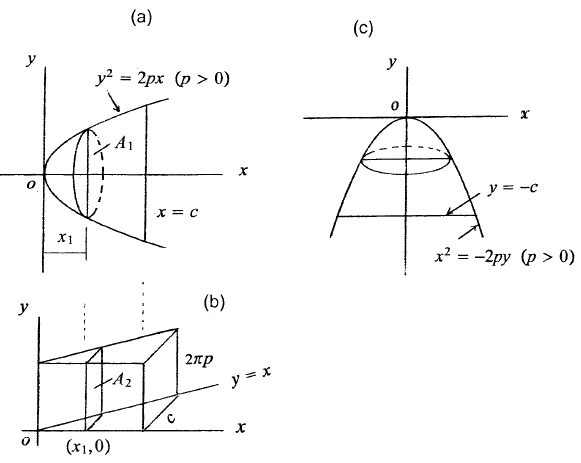A Generalized Cavalieri-Zu Principle
Sidney Kung

# Volume of a Paraboloid of Revolution

We are to find the volume of a solid generated by revolving the region bounded by the parabola $y^{2}=2px$ $(p\gt 0)$ and $x=c$ $(c\gt 0)$ about the $x$-axis. The cross-section of a vertical plane (cutting through point $(0,x_{1})$) and the solid is a circle of radius $\sqrt{2px_{1}}$ produced.Now if we construct a triangular rism of height $2\pi p$, with base area bouned by $y=x$, $x=c$, and the $x$-axis, then the area of the cross-section at $x=x_{1}$ is $A_{2}=2\pi px_{1}$. Hence, by (**), the volume of the solid of revolution is $\frac{1}{2}(c^{2}\cdot 2\pi p)=\pi pc^2$. Similarly, the Volume of a Paraboloid of Revolution by revolving a region bounded by the parabola $x^{2}=-2py$ $(p\gt 0)$ and $y=-c$ $(c\gt 0)$ about the $y$-axis is $\pi pc^2$.Copyright © 1996-2018 Alexander Bogomolny

68545935